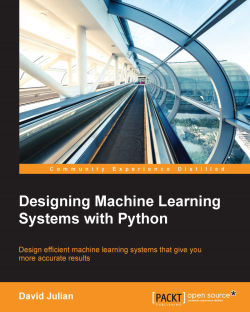### Designing Machine Learning Systems with Python4.2 (5 reviews total)
By David Julian
• \$5 for 5 months Subscribe Access now
• \$35.99 eBook
Advance your knowledge in tech with a Packt subscription

• Instant online access to over 7,500+ books and videos
• Constantly updated with 100+ new titles each month
• Breadth and depth in over 1,000+ technologies

#### About this book

Machine learning is one of the fastest growing trends in modern computing. It has applications in a wide range of fields, including economics, the natural sciences, web development, and business modeling. In order to harness the power of these systems, it is essential that the practitioner develops a solid understanding of the underlying design principles.

There are many reasons why machine learning models may not give accurate results. By looking at these systems from a design perspective, we gain a deeper understanding of the underlying algorithms and the optimisational methods that are available. This book will give you a solid foundation in the machine learning design process, and enable you to build customised machine learning models to solve unique problems. You may already know about, or have worked with, some of the off-the-shelf machine learning models for solving common problems such as spam detection or movie classification, but to begin solving more complex problems, it is important to adapt these models to your own specific needs. This book will give you this understanding and more.

Publication date:
April 2016
Publisher
Packt
Pages
232
ISBN
9781785882951

## Chapter 1. Thinking in Machine Learning

Machine learning systems have a profound and exciting ability to provide important insights to an amazing variety of applications; from groundbreaking and life-saving medical research, to discovering fundamental physical aspects of our universe. From providing us with better, cleaner food, to web analytics and economic modeling. In fact, there are hardly any areas of our lives that have not been touched by this technology in some way. With an expanding Internet of Things, there is a staggering amount of data being generated, and it is clear that intelligent systems are changing societies in quite dramatic ways. With open source tools, such as those provided by Python and its libraries, and the increasing open source knowledge base represented by the Web, it is relatively easy and cheap to learn and apply this technology in new and exciting ways. In this chapter, we will cover the following topics:

• Human interface

• Design principles

• Models

• Unified modelling language

## The human interface

For those of you old enough, or unfortunate enough, to have used early versions of the Microsoft office suite, you will probably remember the Mr Clippy office assistant. This feature, first introduced in Office 97, popped up uninvited from the bottom right-hand side of your computer screen every time you typed the word 'Dear' at the beginning of a document, with the prompt "it looks like you are writing a letter, would you like help with that?".

Mr Clippy, turned on by default in early versions of Office, was almost universally derided by users of the software and could go down in history as one of machine learning's first big fails.

So, why was the cheery Mr Clippy so hated? Clearly the folks at Microsoft, at the forefront of consumer software development, were not stupid, and the idea that an automated assistant could help with day to day office tasks is not necessarily a bad idea. Indeed, later incarnations of automated assistants, the best ones at least, operate seamlessly in the background and provide a demonstrable increase in work efficiency. Consider predictive text. There are many examples, some very funny, of where predictive text has gone spectacularly wrong, but in the majority of cases where it doesn't fail, it goes unnoticed. It just becomes part of our normal work flow.

At this point, we need a distinction between error and failure. Mr Clippy failed because it was obtrusive and poorly designed, not necessarily because it was in error; that is, it could make the right suggestion, but chances are you already know that you are writing a letter. Predictive text has a high error rate, that is, it often gets the prediction wrong, but it does not fail largely because of the way it is designed to fail: unobtrusively.

The design of any system that has a tightly coupled human interface, to use systems engineering speak, is difficult. Human behavior, like the natural world in general, is not something we can always predict. Expression recognition systems, natural language processing, and gesture recognition technology, amongst other things, all open up new ways of human-machine interaction, and this has important applications for the machine learning specialist.

Whenever we are designing a system that requires human input, we need to anticipate the possible ways, not just the intended ways, a human will interact with the system. In essence, what we are trying to do with these systems is to instil in them some understanding of the broad panorama of human experience.

In the first years of the web, search engines used a simple system based on the number of times search terms appeared in articles. Web developers soon began gaming the system by increasing the number of key search terms. Clearly, this would lead to a keyword arms race and result in a very boring web. The page rank system measuring the number of quality inbound links was designed to provide a more accurate search result. Now, of course, modern search engines use more sophisticated and secret algorithms.

What is also important for ML designers is the ever increasing amount of data that is being generated. This presents several challenges, most notably its sheer vastness. However, the power of algorithms in extracting knowledge and insights that would not have been possible with smaller data sets is massive. So, many human interactions are now digitized, and we are only just beginning to understand and explore the many ways in which this data can be used.

As a curious example, consider the study The expression of emotion in 20th century books (Acerbi et al, 2013). Though strictly more of a data analysis study, rather than machine learning, it is illustrative for several reasons. Its purpose was to chart the emotional content, in terms of a mood score, of text extracted from books of the 20th century. With access to a large volume of digitized text through the project Gutenberg digital library, WordNet (http://wordnet.princeton.edu/wordnet/), and Google's Ngram database (books.google.com/ngrams), the authors of this study were able to map cultural change over the 20th century as reflected in the literature of the time. They did this by mapping trends in the usage of the mood words.

For this study, the authors labeled each word (a 1gram) and associated it with a mood score and the year it was published. We can see that emotion words, such as joy, sadness, fear, and so forth, can be scored according to the positive or negative mood they evoke. The mood score was obtained from WordNet (wordnet.princeton.edu). WordNet assigns an affect score to each mood word. Finally, the authors simply counted the occurrences of each mood word: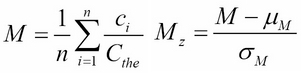Here, ci is the count of a particular mood word, n is the total count of mood words (not all words, just words with a mood score), and Cthe is the count of the word the in the text. This normalizes the sum to take into account that some years more books were written (or digitized). Also, since many later books tend to contain more technical language, the word the was used to normalize rather than get the total word count. This gives a more accurate representation of emotion over a long time period in prose text. Finally, the score is normalized according to a normal distribution, Mz, by subtracting the mean and dividing by the standard deviation.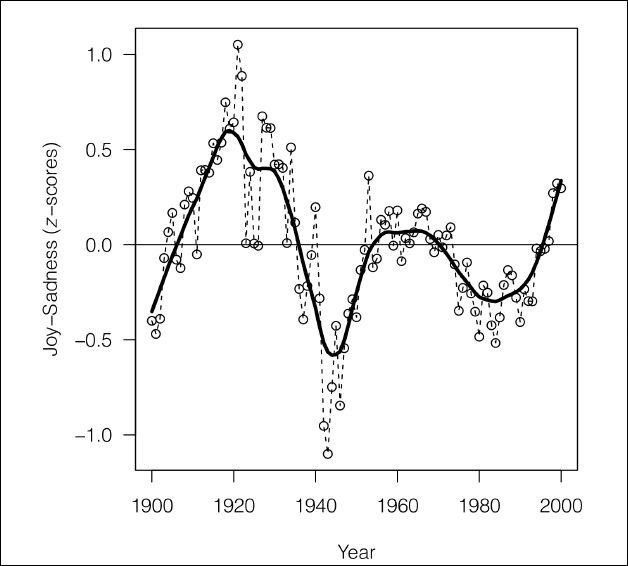This figure is taken from The expression of Emotions in 20th Century Books, (Alberto Acerbi, Vasileios Lampos, Phillip Garnett, R. Alexander Bentley) PLOS.

Here we can see one of the graphs generated by this study. It shows the joy-sadness score for books written in this period, and clearly shows a negative trend associated with the period of World War II.

This study is interesting for several reasons. Firstly, it is an example of data-driven science, where previously considered soft sciences, such as sociology and anthropology, are given a solid empirical footing. Despite some pretty impressive results, this study was relatively easy to implement. This is mainly because most of the hard work had already been done by WordNet and Google. This highlights how using data resources that are freely available on the Internet, and software tools such as the Python's data and machine learning packages, anyone with the data skills and motivation can build on this work.

## Design principles

An analogy is often made between systems design and designing other things such as a house. To a certain extent, this analogy holds true. We are attempting to place design components into a structure that meets a specification. The analogy breaks down when we consider their respective operating environments. It is generally assumed in the design of a house that the landscape, once suitably formed, will not change.

Software environments are slightly different. Systems are interactive and dynamic. Any system that we design will be nested inside other systems, either electronic, physical, or human. In the same way different layers in computer networks (application layer, transport layer, physical layer, and so on) nest different sets of meanings and function, so to do activities performed at different levels of a project.

As the designer of these systems, we must also have a strong awareness of the setting, that is, the domain in which we work. This knowledge gives us clues to patterns in our data and helps us give context to our work.

Machine learning projects can be divided into five distinct activities, shown as follows:

• Defining the object and specification

• Preparing and exploring the data

• Model building

• Implementation

• Testing

• Deployment

The designer is mainly concerned with the first three. However, they often play, and in many projects must play, a major role in other activities. It should also be said that a project's timeline is not necessarily a linear sequence of these activities. The important point is that they are distinct activities. They may occur in parallel to each other, and in other ways interact with each other, but they generally involve different types of tasks that can be separated in terms of human and other resources, the stage of the project, and externalities. Also, we need to consider that different activities involve distinct operational modes. Consider the different ways in which your brain works when you are sketching out an idea, as compared to when you are working on a specific analytical task, say a piece of code.

Often, the hardest question is where to begin. We can start drilling into the different elements of a problem, with an idea of a feature set and perhaps an idea of the model or models we might use. This may lead to a defined object and specification, or we may have to do some preliminary research such as checking possible data sets and sources, available technologies, or talking to other engineers, technicians, and users of the system. We need to explore the operating environment and the various constraints; is it part of a web application, or is it a laboratory research tool for scientists?

In the early stages of design, our work flow will flip between working on the different elements. For instance, we start with a general problem—perhaps having an idea of the task, or tasks, necessary to solve it—then we divide it into what we think are the key features, try it out on a few models with a toy dataset, go back to refine the feature set, adjust our model, precisely define tasks, and refine the model. When we feel our system is robust enough, we can test it out on some real data. Of course, then we may need to go back and change our feature set.

Selecting and optimizing features is often a major activity (really, a task in itself) for the machine learning designer. We cannot really decide what features we need until we have adequately described the task, and of course, both the task and features are constrained by the types of feasible models we can build.

### Types of questions

As designers, we are asked to solve a problem. We are given some data and an expected output. The first step is to frame the problem in a way that a machine can understand it, and in a way that carries meaning for a human. The following six broad approaches are what we can take to precisely define our machine learning problem:

• Exploratory: Here, we analyze data, looking for patterns such as a trend or relationship between variables. Exploration will often lead to a hypothesis such as linking diet with disease, or crime rate with urban dwellings.

• Descriptive: Here, we try to summarize specific features of our data. For instance, the average life expectancy, average temperature, or the number of left-handed people in a population.

• Inferential: An inferential question is one that attempts to support a hypothesis, for instance, proving (or disproving) a general link between life expectancy and income by using different data sets.

• Predictive: Here, we are trying to anticipate future behavior. For instance, predicting life expectancy by analyzing income.

• Casual: This is an attempt to find out what causes something. Does low income cause a lower life expectancy?

• Mechanistic: This tries to answer questions such as "what are the mechanisms that link income with life expectancy?"

Most machine learning problems involve several of these types of questions during development. For instance, we may first explore the data looking for patterns or trends, and then we may describe certain key features of our data. This may enable us to make a prediction, and find a cause or a mechanism behind a particular problem.

### Are you asking the right question?

The question must be plausible and meaningful in its subject area. This domain knowledge enables you to understand the things that are important in your data and to see where a certain pattern or correlation has meaning.

The question should be as specific as possible, while still giving a meaningful answer. It is common for it to begin as a generalized statement, such as "I wonder if wealthy means healthy". So, you do some further research and find you can get statistics for wealth by geographic region, say from the tax office. We can measure health through its inverse, that is, illness, say by hospital admissions, and we can test our initial proposition, "wealthy means healthy", by tying illness to geographic region. We can see that a more specific question relies on several, perhaps questionable, assumptions.

We should also consider that our results may be confounded by the fact that poorer people may not have healthcare insurance, so are less likely to go to a hospital despite illness. There is an interaction between what we want to find out and what we are trying to measure. This interaction perhaps hides a true rate of illness. All is not lost, however. Because we know about these things, then perhaps we can account for them in our model.

We can make things a lot easier by learning as much as we can about the domain we are working in.

You could possibly save yourself a lot of time by checking whether the question you are asking, or part of it, has already been answered, or if there are data sets available that may shed some light on that topic. Often, you have to approach a problem from several different angles at once. Do as much preparatory research as you can. It is quite likely that other designers have done work that could shed light on your own.

### Tasks

A task is a specific activity conducted over a period of time. We have to distinguish between the human tasks (planning, designing, and implementing) to the machine tasks (classification, clustering, regression, and so on). Also consider when there is overlap between human and machine, for example, as in selecting features for a model. Our true goal in machine learning is to transform as many of these tasks as we can from human tasks to machine tasks.

It is not always easy to match a real world problem to a specific task. Many real world problems may seem to be conceptually linked but require a very different solution. Alternatively, problems that appear completely different may require similar methods. Unfortunately, there is no simple lookup table to match a particular task to a problem. A lot depends on the setting and domain. A similar problem in one domain may be unsolvable in another, perhaps because of lack of data. There are, however, a small number of tasks that are applied to a large number of methods to solve many of the most common problem types. In other words, in the space of all possible programming tasks, there is a subset of tasks that are useful to our particular problem. Within this subset, there is a smaller subset of tasks that are easy and can actually be applied usefully to our problem.

Machine learning tasks occur in three broad settings:

• Supervised learning: The goal here is to learn a model from labeled training data that allows predictions to be made on unseen future data.

• Unsupervised learning: Here we deal with unlabeled data and our goal is to find hidden patterns in this data to extract meaningful information.

• Reinforcement learning: The goal here is to develop a system that improves its performance based on the interactions it has with its environment. This usually involves a reward signal. This is similar to supervised learning, except that rather than having a labeled training set, reinforcement learning uses a reward function to continually improve its performance.

Now, let's take a look at some of the major machine learning tasks. The following diagram should give you a starting point to try and decide what type of task is appropriate for different machine learning problems: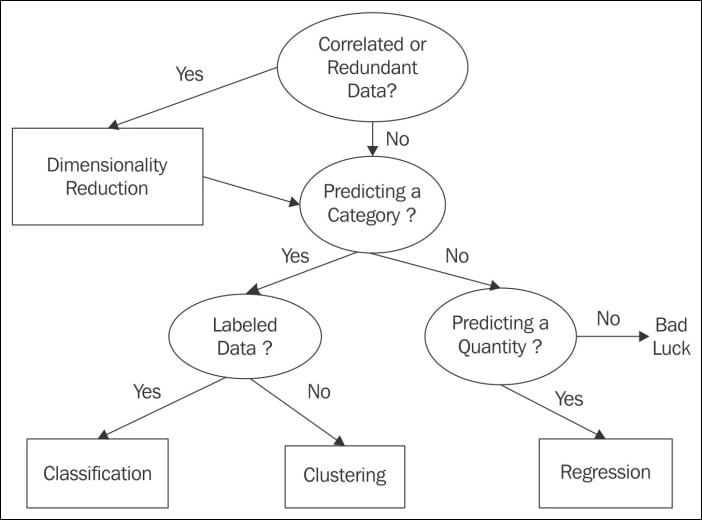#### Classification

Classification is probably the most common type of task; this is due in part to the fact that it is relatively easy, well understood, and solves a lot of common problems. Classification is about assigning classes to a set of instances, based on their features. This is a supervised learning method because it relies on a labeled training set to learn a set of model parameters. This model can then be applied to unlabeled data to make a prediction on what class each instance belongs to. There are broadly two types of classification tasks: binary classification and multiclass classification. A typical binary classification task is e-mail spam detection. Here we use the contents of an e-mail to determine if it belongs to one of the two classes: spam or not spam. An example of multiclass classification is handwriting recognition, where we try to predict a class, for example, the letter name. In this case, we have one class for each of the alpha numeric characters. Multiclass classification can sometimes be achieved by chaining binary classification tasks together, however, we lose information this way, and we are unable to define a single decision boundary. For this reason, multiclass classification is often treated separately from binary classification.

#### Regression

There are cases where what we are interested in are not discrete classes, but a continuous variable, for instance, a probability. These types of problems are regression problems. The aim of regression analysis is to understand how changes to the input, independent variables, effect changes to the dependent variable. The simplest regression problems are linear and involve fitting a straight line to a set of data in order to make a prediction. This is usually done by minimizing the sum of squared errors in each instance in the training set. Typical regression problems include estimating the likelihood of a disease given a range and severity of symptoms, or predicting test scores given past performance.

#### Clustering

Clustering is the most well known unsupervised method. Here, we are concerned with making a measurement of similarity between instances in an unlabeled dataset. We often use geometric models to determine the distance between instances, based on their feature values. We can use an arbitrary measurement of closeness to determine what cluster each instance belongs to. Clustering is often used in data mining and exploratory data analysis. There are a large variety of methods and algorithms that perform this task, and some of the approaches include the distance-based method, as well as finding a center point for each cluster, or using statistical techniques based on distributions.

Related to clustering is association; this is an unsupervised task to find a certain type of pattern in the data. This task is behind product recommender systems such as those provided by Amazon and other on-line shops.

#### Dimensionality reduction

Many data sets contain a large number of features or measurements associated with each instance. This can present a challenge in terms of computational power and memory allocation. Also many features may contain redundant information or information that is correlated to other features. In these cases, the performance of our learning model may be significantly degraded. Dimensionality reduction is most often used in feature prepossessing; it compresses the data into a lower dimension sub space while retaining useful information. Dimensionality reduction is also used when we want to visualize data, typically by projecting higher dimensions onto one, two, or three dimensions.

From these basic machine tasks, there are a number of derived tasks. In many applications, this may simply be applying the learning model to a prediction to establish a casual relationship. We must remember that explaining and predicting are not the same. A model can make a prediction, but unless we know explicitly how it made the prediction, we cannot begin to form a comprehensible explanation. An explanation requires human knowledge of the domain.

We can also use a prediction model to find exceptions from a general pattern. Here we are interested in the individual cases that deviate from the predictions. This is often called anomaly detection and has wide applications in things like detecting bank fraud, noise filtering, and even in the search for extraterrestrial life.

An important and potentially useful task is subgroup discovery. Our goal here is not, as in clustering, to partition the entire domain, but rather to find a subgroup that has a substantially different distribution. In essence, subgroup discovery is trying to find relationships between a dependent target variables and many independent explaining variables. We are not trying to find a complete relationship, but rather a group of instances that are different in ways that are important to the domain. For instance, establishing a subgroup, smoker = true and family history = true for a target variable of heart disease = true.

Finally, we consider control type tasks. These act to optimize control settings to maximize a payoff, given different conditions. This can be achieved in several ways. We can clone expert behavior: the machine learns directly from a human and makes predictions on actions given different conditions. The task is to learn a prediction model for the expert's actions. This is similar to reinforcement learning, where the task is to learn a relationship between conditions and optimal action.

#### Errors

In machine learning systems, software flaws can have very serious real world consequences; what happens if your algorithm, embedded in an assembly line robot, classifies a human as a production component? Clearly, in critical systems, you need to plan for failure. There should be a robust fault and error detection procedure embedded in your design process and systems.

Sometimes it is necessary to design very complex systems simply for the purpose of debugging and checking for logic flaws. It may be necessary to generate data sets with specific statistical structures, or create artificial humans to mimic an interface. For example, developing a methodology to verify that the logic of your design is sound at the data, model, and task levels. Errors can be hard to track, and as a scientist, you must assume that there are errors and try to prove otherwise.

The idea of recognizing and gracefully catching errors is important for the software designer, but as machine learning systems designers, we must take it a step further. We need to be able to capture, in our models, the ability to learn from an error.

Consideration must be given to how we select our test set, and in particular, how representative it is of the rest of the dataset. For instance, if it is noisy compared to the training set, it will give poor results on the test set, suggesting that our model is overfitting, when in fact, this is not the case. To avoid this, a process of cross validation is used. This works by randomly dividing the data into, for example, ten chunks of equal size. We use nine chunks for training the model and one for testing. We do this 10 times, using each chunk once for testing. Finally, we take an average of test set performance. Cross validation is used with other supervised learning problems besides classification, but as you would expect, unsupervised learning problems need to be evaluated differently.

With an unsupervised task we do not have a labeled training set. Evaluation can therefore be a little tricky since we do not know what a correct answer looks like. In a clustering problem, for instance, we can compare the quality of different models by measures such as the ratio of cluster diameter compared to the distance between clusters. However, in problems of any complexity, we can never tell if there is another model, not yet built, which is better.

#### Optimization

Optimization problems are ubiquitous in many different domains, such as finance, business, management, sciences, mathematics, and engineering. Optimization problems consist of the following:

• An objective function that we want to maximize or minimize.

• Decision variables, that is, a set of controllable inputs. These inputs are varied within the specified constraints in order to satisfy the objective function.

• Parameters, which are uncontrollable or fixed inputs.

• Constraints are relations between decision variables and parameters. They define what values the decision variables can have.

Most optimization problems have a single objective function. In the cases where we may have multiple objective functions, we often find that they conflict with each other, for example, reducing costs and increasing output. In practice, we try to reformulate multiple objectives into a single function, perhaps by creating a weighted combination of objective functions. In our costs and output example, a variable along the lines of cost per unit might work.

The decision variables are the variables we control to achieve the objective. They may include things such as resources or labor. The parameters of the module are fixed for each run of the model. We may use several cases, where we choose different parameters to test variations in multiple conditions.

There are literally thousands of solution algorithms to the many different types of optimization problems. Most of them involve first finding a feasible solution, then iteratively improving on it by adjusting the decision variables to hopefully find an optimum solution. Many optimization problems can be solved reasonably well with linear programming techniques. They assume that the objective function and all the constraints are linear with respect to the decision variables. Where these relationships are not linear, we often use a suitable quadratic function. If the system is non-linear, then the objective function may not be convex. That is, it may have more than one local minima, and there is no assurance that a local minima is a global minima.

#### Linear programming

Why are linear models so ubiquitous? Firstly, they are relatively easy to understand and implement. They are based on a well founded mathematical theory that was developed around the mid 1700s and that later played a pivotal role in the development of the digital computer. Computers are uniquely tasked to implement linear programs because computers were conceptualized largely on the basis of the theory of linear programming. Linear functions are always convex, meaning they have only one minima. Linear Programming (LP) problems are usually solved using the simplex method. Suppose that we want to solve the optimization problem, we would use the following syntax:

max x1 + x2 with constraints: 2x1 + x2 ≤ 4 and x1 + 2x2 ≤ 3

We assume that x1 and x2 are greater than or equal to 0. The first thing we need to do is convert it to the standard form. This is done by ensuring the problem is a maximization problem, that is, we convert min z to max -z. We also need to convert the inequalities to equalities by adding non-negative slack variables. The example here is already a maximization problem, so we can leave our objective function as it is. We do need to change the inequalities in the constraints to equalities:

2x1 + x2 + x3 = 4 and x1 + 2x2 + x4 = 3

If we let z cf the objective function, we can then write the following:

z - x1 - x2 = 0

We now have the following system of linear equations:

• Objective: z - x1 - x2 + 0 + 0 = 0

• Constraint 1: 2x1 + x2 + x3 + 0 = 4

• Constraint 2: x1 + 2x2 + 0 + x4 = 3

Our objective is to maximize z, remembering that all variables are non-negative. We can see that x1 and x2 appear in all the equations and are called non-basic. The x3 and x4 value only appear in one equation each. They are called basic variables. We can find a basic solution by assigning all non-basic variables to 0. Here, this gives us the following:

x1 = x2 = 0; x3 = 4; x4 = 3; z = 0

Is this an optimum solution, remembering that our goal is to maximize z? We can see that since z subtracts x1 and x2 in the first equation in our linear system, we are able to increase these variables. If the coefficients in this equation were all non-negative, then there would be no way to increase z. We will know that we have found an optimum solution when all coefficients in the objective equation are positive.

This is not the case here. So, we take one of the non-basic variables with a negative coefficient in the objective equation (say x1, which is called the entering variable) and use a technique called pivoting to turn it from a non-basic to a basic variable. At the same time, we will change a basic variable, called the leaving variable, into a non-basic one. We can see that x1 appears in both the constraint equations, so which one do we choose to pivot? Remembering that we need to keep the coefficients positive. We find that by using the pivot element that yields the lowest ratio of right-hand side of the equations to their respective entering coefficients, we can find another basic solution. For x1, in this example, it gives us 4/2 for the first constraint and 3/1 for the second. So, we will pivot using x1 in constraint 1.

We divide constraint 1 by 2, and get the following:

x1 + ½ x2 + ½x3 = 2

We can now write this in terms of x1, and substitute it into the other equations to eliminate x1 from those equations. Once we have performed a bit of algebra, we end up with the following linear system:

z - 1/2x2 + 1/3 x3 = 2

x1 + 1/2 x2 + 1/2x3 = 2

3/2x2 – 1/2x3 + x4 = 1

We have another basic solution. But, is this the optimal solution? Since we still have a minus coefficient in the first equation, the answer is no. We can now go through the same pivot process with x2, and using the ratio rule, we find that we can pivot on 3/2x2 in the third equation. This gives us the following:

z + 1/3x3 + 1/3x4 = 7/3

x1 + 2/3x3 - 1/3 x4 = 5/3

x2 - 1/3x3 + 2/3 x4 = 2/3

This gives us the solution to x3 = x4 = 0, x1 = 5/3, x2 = 2/3, and z = 7/3. This is the optimal solution because there are no more negatives in the first equation.

We can visualize this with the following graph. The shaded area is the region where we will find a feasible solution: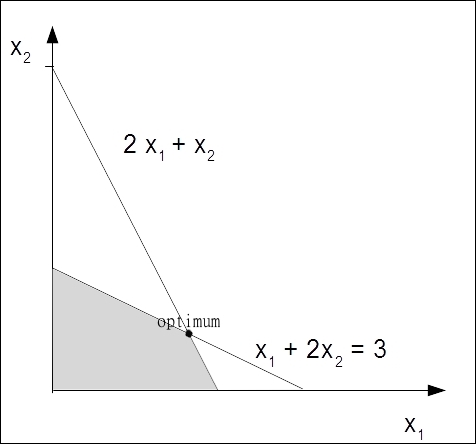The two variable optimization problem

#### Models

Linear programming gives us a strategy for encoding real world problems into the language of computers. However, we must remember that our goal is not to just solve an instance of a problem, but to create a model that will solve unique problems from new data. This is the essence of learning. A learning model must have a mechanism to evaluate its output, and in turn, change its behavior to a state that is closer to a solution.

The model is essentially a hypothesis, that is, a proposed explanation of a phenomena. The goal is for it to apply a generalization to the problem. In the case of a supervised learning problem, knowledge gained from the training set is applied to the unlabeled test. In the case of an unsupervised learning problem, such as clustering, the system does not learn from a training set. It must learn from the characteristics of the data set itself, such as the degree of similarity. In both cases, the process is iterative. It repeats a well-defined set of tasks, which moves the model closer to a correct hypothesis.

Models are the core of a machine learning system. They are what does the learning. There are many models, with as many variations on these models, as there are unique solutions. We can see that the problems machine learning systems solve (regression, classification, association, and so on) come up in many different settings. They have been used successfully in almost all branches of science, engineering, mathematics, commerce, and also in the social sciences; they are as diverse as the domains they operate in.

This diversity of models gives machine learning systems great problem solving power. However, it can also be a bit daunting for the designer to decide which is the best model, or models, are for a particular problem. To complicate things, there are often several models that may solve your task, or your task may need several models. Which is the most accurate and efficient pathway through an original problem is something you simply cannot know when you embark upon such a project.

For our purposes here, let's break this broad canvas into three overlapping, non-mutual, and exclusive categories: geometric, probabilistic, and logical. Within these three models, a distinction must be made regarding how a model divides up the instance space. The instance space can be considered as all the possible instances of your data, regardless of whether each instance appears in the data. The actual data is a subset of the space of the instance space.

There are two approaches to dividing up this space: grouping and grading. The key difference between the two is that grouping models divide the instance space into fixed discrete units called segments. They have a finite resolution and cannot distinguish between classes beyond this resolution. Grading, on the other hand, forms a global model over the entire instance space, rather than dividing the space into segments. In theory, their resolution is infinite, and they can distinguish between instances no matter how similar they are. The distinction between grouping and grading is not absolute, and many models contain elements of both. For instance, a linear classifier is generally considered a grading model because it is based on a continuous function. However, there are instances that the linear model cannot distinguish between, for example, a line or surface parallel to the decision boundary.

##### Geometric models

Geometric models use the concept of instance space. The most obvious example of geometric models is when all the features are numerical and can become coordinates in a Cartesian coordinate system. When we only have two or three features, they are easy to visualize. However, since many machine learning problems have hundreds or thousands of features, and therefore dimensions, visualizing these spaces is impossible. However, many of the geometric concepts, such as linear transformations, still apply in this hyper space. This can help us better understand our models. For instance, we expect that many learning algorithms will be translation invariant, that is, it does not matter where we place the origin in the coordinate system. Also, we can use the geometric concept of Euclidean distance to measure any similarities between instances; this gives us a method to cluster like instances and form a decision boundary between them.

Supposing we are using our linear classifier to classify paragraphs as either happy or sad and we have devised a set of tests. Each test is associated with a weight, w, to determine how much each test contributes to the overall result.

We can simply sum up each test and multiply it by its weight to get an overall score and create a decision rule that will create a boundary, for example, if the happy score is greater than a threshold, t.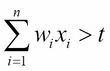Each feature contributes independently to the overall result, hence the rules linearity. This contribution depends on each feature's relative weight. This weight can be positive or negative, and each individual feature is not subject to the threshold while calculating the overall score.

We can rewrite this sum with vector notation using w for a vector of weights (w1, w2, ..., wn) and x for a vector of test results (x1, x2, ..., xn). Also, if we make it an equality, we can define the decision boundary:

w . x = t

We can think of w as a vector pointing between the "centers of mass" of the positive (happy) examples, P, and the negative examples, N. We can calculate these centers of mass by averaging the following: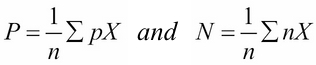Our aim now is to create a decision boundary half way between these centers of mass. We can see that w is proportional, or equal, to P - N, and that (P + N)/2 will be on the decision boundary. So, we can write the following: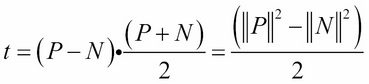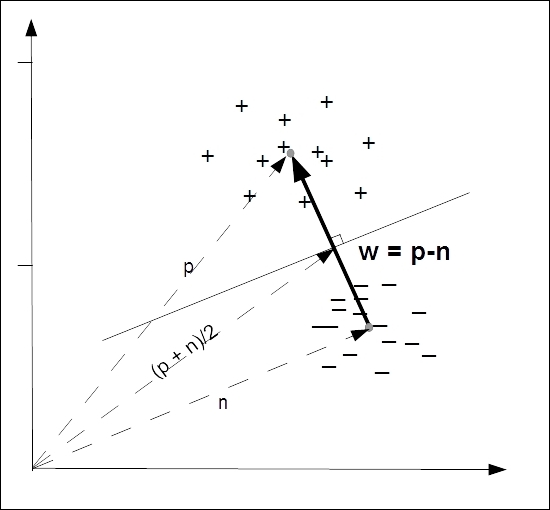Fig of Decision boundary

In practice, real data is noisy and not necessarily that is easy to separate. Even when data is easily separable, a particular decision boundary may not have much meaning. Consider data that is sparse, such as in text classification where the number of words is large compared to the number of instances of each word. In this large area of empty instance space, it may be easy to find a decision boundary, but which is the best one? One way to choose is to use a margin to measure the distance between the decision boundary and its closest instance. We will explore these techniques later in the book.

##### Probabilistic models

A typical example of a probabilistic model is the Bayesian classifier, where you are given some training data (D), and a probability based on an initial training set (a particular hypothesis, h), getting the posteriori probability, P (h/D).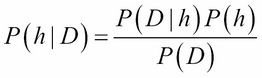As an example, consider that we have a bag of marbles. We know that 40 percent of them are red and 60 percent are blue. We also know that half of the red marbles and all the blue marbles have flecks of white. When we reach into the bag to select a marble, we can feel by its texture that it has flecks. What are the chances of it being red?

Let P(RF) be equal to the probability that a randomly drawn marble with flecks is red:

P(FR) = the probability of a red marble with flecks is 0.5.

P(R) = the probability a marble being red is 0.4.

P(F) = the probability that a marble has flecks is 0.5 x 0.4 + 1 x 0.6= 0.8.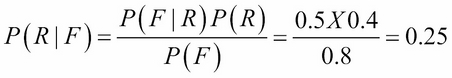Probabilistic models allow us to explicitly calculate probabilities, rather than just a binary true or false. As we know, the key thing we need to do is create a model that maps or features a variable to a target variable. When we take a probabilistic approach, we assume that there is an underlying random process that creates a well defined but unknown probability distribution.

Consider a spam detector. Our feature variable, X, could consist of a set of words that indicate the email might be spam. The target variable, Y, is the instance class, either spam or ham. We are interested in the conditional probability of Y given X. For each email instance, there will be a feature vector, X, consisting of Boolean values representing the presence of our spam words. We are trying to find out whether Y, our target Boolean, is representing spam or not spam.

Now, consider that we have two words, x1 and x2, that constitute our feature vector X. From our training set, we can construct a table such as the following one:

P(Y = spam| x1, x2)

P(Y = not spam| x1, x2)

P(Y| x1 = 0, x2 = 0)

0.1

0.9

P(Y| x1 = 0, x2 = 1)

0.7

0.3

P(Y| x1 = 1, x2 = 0)

0.4

0.6

P(Y| x1 = 1, x2 = 1)

0.8

0.2

Table 1.1

We can see that once we begin adding more words to our feature vector, it will quickly grow unmanageable. With a feature vector of n size, we will have 2n cases to distinguish. Fortunately, there are other methods to deal with this problem, as we shall see later.

The probabilities in the preceding table are known as posterior probabilities. These are used when we have knowledge from a prior distribution. For instance, that one in ten emails is spam. However, consider a case where we may know that X contains x2 = 1, but we are unsure of the value of x1. This instance could belong in row 2, where the probability of it being spam is 0.7, or in row 4, where the probability is 0.8. The solution is to average these two rows using the probability of x1 = 1 in any instance. That is, the probability that a word, x1, will appear in any email, spam or not:

P(Y|x2 = 1) = P(Y|x1 = 0, x2 = 1)P(x1 = 0) + P(x1 = 1,x2 = 1)P(x1 = 1)

This is called a likelihood function. If we know, from a training set, that the probability that x1 is one is 0.1 then the probability that it is zero is 0.9 since these probabilities must sum to 1. So, we can calculate the probability that an e-mail contains the spam word 0.7 * 0.9 + 0.8 * 0.1 = 0.71.

This is an example of a likelihood function: P(X|Y). So, why do we want to know the probability of X, which is something we all ready know, conditioned on Y, which is something we know nothing about? A way to look at this is to consider the probability of any email containing a particular random paragraph, say, the 127th paragraph of War and Peace. Clearly, this probability is small, regardless of whether the e-mail is spam or not. What we are really interested in is not the magnitude of these likelihoods, but rather their ratio. How much more likely is an email containing a particular combination of words to be spam or not spam? These are sometimes called generative models because we can sample across all the variables involved.

We can use Bayes' rule to transform between prior distributions and a likelihood function: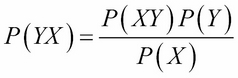P(Y) is the prior probability, that is, how likely each class is, before having observed X. Similarly, P(X) is the probability without taking into account Y. If we have only two classes, we can work with ratios. For instance, if we want to know how much the data favors each class, we can use the following: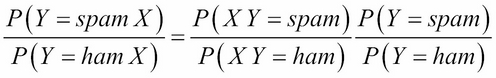If the odds are less than one, we assume that the class in the denominator is the most likely. If it is greater than one, then the class in the enumerator is the most likely. If we use the data from Table 1.1, we calculate the following posterior odds: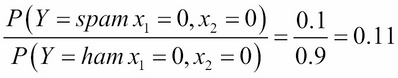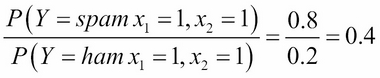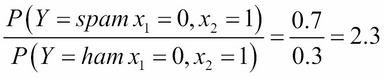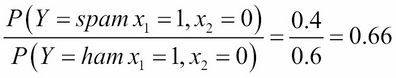The likelihood function is important in machine learning because it creates a generative model. If we know the probability distribution of each word in a vocabulary, together with the likelihood of each one appearing in either a spam or not spam e-mail, we can generate a random spam e-mail according to the conditional probability, P(X|Y = spam).

##### Logical models

Logical models are based on algorithms. They can be translated into a set of formal rules that can be understood by humans. For example, if both x1 and x2 are 1 then the email is classified as spam.

These logical rules can be organized into a tree structure. In the following figure, we see that the instance space is iteratively partitioned at each branch. The leaves consist of rectangular areas (or hyper rectangles in the case of higher dimensions) representing segments of the instance space. Depending on the task we are solving, the leaves are labeled with a class, probability, real number, and so on.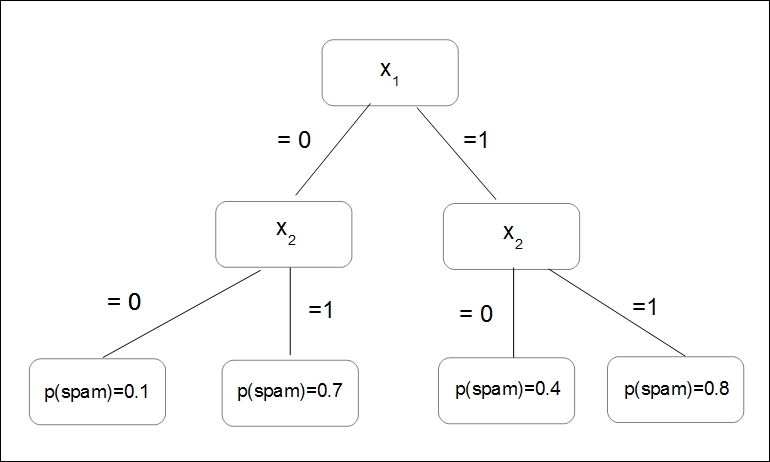The figure feature tree

Feature trees are very useful when representing machine learning problems; even those that, at first sight, do not appear to have a tree structure. For instance, in the Bayes classifier in the previous section, we can partition our instance space into as many regions as there are combinations of feature values. Decision tree models often employ a pruning technique to delete branches that give an incorrect result. In Chapter 3, Turning Data into Information, we will look at a number of ways to represent decision trees in Python.

### Note

Note that decision rules may overlap and make contradictory predictions.

They are then said to be logically inconsistent. Rules can also be incomplete when they do not take into account all the coordinates in the feature space. There are a number of ways that we can address these issues, and we will look at these in detail later in the book.

Since tree learning algorithms usually work in a top down manner, the first task is to find a good feature to split on at the top of the tree. We need to find a split that will result in a higher degree of purity in subsequent nodes. By purity, I mean the degree to which training examples all belong to the same class. As we descend down the tree, at each level, we find the training examples at each node increase in purity, that is, they increasingly become separated into their own classes until we reach the leaf where all examples belong to the same class.

To look at this in another way, we are interested in lowering the entropy of subsequent nodes in our decision tree. Entropy, a measure of disorder, is high at the top of the tree (the root) and is progressively lowered at each node as the data is divided up into its respective classes.

In more complex problems, those with larger feature sets and decision rules, finding the optimum splits is sometimes not possible, at least not in an acceptable amount of time. We are really interested in creating the shallowest tree to reach our leaves in the shortest path. In the time it takes to analyze, each node grows exponentially with each additional feature, so the optimum decision tree may take longer to find than actually using a sub-optimum tree to perform the task.

An important property of logical models is that they can, to some extent, provide an explanation for their predictions. For example, consider the predictions made by a decision tree. By tracing the path from leaf to root we can determine the conditions that resulted in the final result. This is one of the advantages of logical models: they can be inspected by a human to reveal more about the problem.

#### Features

In the same way that decisions are only as good as the information available to us in real life, in a machine learning task, the model is only as good as its features. Mathematically, features are a function that maps from the instance space to a set of values in a particular domain. In machine learning, most measurements we make are numerical, and therefore the most common feature domain is the set of real numbers. Other common domains include Boolean, true or false, integers (say, when we are counting the occurrence of a particular feature), or finite sets such as a set of colors or shapes.

Models are defined in terms of their features. Also, single features can be turned into a model, which is known as a univariate model. We can distinguish between two uses of features. This is related to the distinction between grouping and grading.

Firstly, we can group our features by zooming into an area in the instance space. Let f be a feature counting the number of occurrences of a word, x1, in an e-mail, X. We can set up conditions such as the following:

Where f(X)=0, representing emails that do not contain x1 or where f(X)>0 representing emails that contain x1 one or more times. These conditions are called binary splits because they divide the instance space into two groups: those that satisfy the condition and those that don't. We can also split the instance space into more than two segments to create non-binary splits. For instance, where f(X) = 0; 0 < F(X) < 5; F(X) > 5, and so on.

Secondly, we can grade our features to calculate the independent contribution each one makes to the overall result. Recall our simple linear classifier, the decision rule of the following form: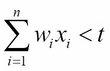Since this rule is linear, each feature makes an independent contribution to the score of an instance. This contribution depends on wi. If it is positive, then a positive xi will increase the score. If wi is negative, a positive xi decreases the score. If wi is small or zero, then the contribution it makes to the overall result is negligible. It can be seen that the features make a measurable contribution to the final prediction.

These two uses of features, as splits (grouping) and predictors (grading), can be combined into one model. A typical example occurs when we want to approximate a non-linear function, say y sin π x, on the interval, -1 < x < 1. Clearly, the simple linear model will not work. Of course, the simple answer is to split the x axis into -1 < x 0 and 0 <. On each of these segments, we can find a reasonable linear approximation.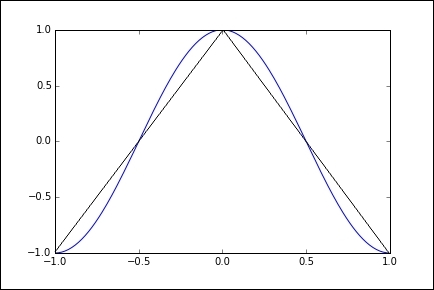Using grouping and grading

A lot of work can be done to improve our model's performance by feature construction and transformation. In most machine learning problems, the features are not necessarily explicitly available. They need to be constructed from raw datasets and then transformed into something that our model can make use of. This is especially important in problems such as text classification. In our simple spam example, we used what is known as a bag of words representation because it disregards the order of the words. However, by doing this, we lose important information about the meaning of the text.

An important part of feature construction is discretization. We can sometimes extract more information, or information that is more relevant to our task, by dividing features into relevant chunks. For instance, supposing our data consists of a list of people's precise incomes, and we are trying to determine whether there is a relationship between financial income and the suburb a person lives in. Clearly, it would be appropriate if our feature set did not consist of precise incomes but rather ranges of income, although strictly speaking, we would lose information. If we choose our intervals appropriately, we will not lose information related to our problem, and our model will perform better and give us results that are easier to interpret.

This highlights the major tasks of feature selection: separating the signal from the noise.

Real world data will invariably contain a lot of information that we do not need, as well as just plain random noise, and separating the, perhaps small, part of the data that is relevant to our needs is important to the success of our model. It is of course important that we do not throw out information that may be important to us.

Often, our features will be non-linear, and linear regression may not give us good results. A trick is to transform the instance space itself. Supposing we have data such as what is shown in the following figure. Clearly, linear regression only gives us a reasonable fit, as shown in the figure on the left-hand side. However, we can improve this result if we square the instance space, that is, we make x = x2 and y = y2, as shown in the figure on the right-hand side: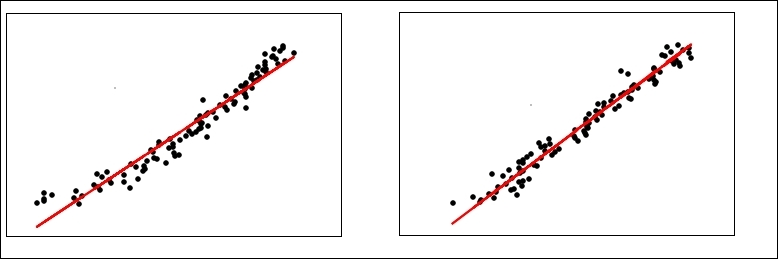Variance = .92      Variance = .97

Transforming the instance space

We can go further and use a technique called the kernel trick. The idea is that we can create a higher dimensional implicit feature space. Pairs of data points are mapped from the raw dataset to this higher dimensional space via a specified function, sometimes called a similarity function.

For instance, let x1 = (x1, y1) and x2 = (x2, y2).

We create a 2D to 3D mapping, shown as follows: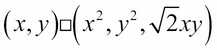The points in the 3D space corresponding to the 2D points, x1 and x2, are as follows: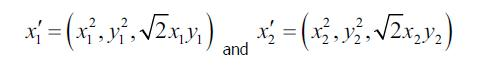Now, the dot product of these two vectors is: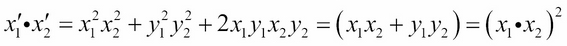We can see that by squaring the dot product in the original 2D space, we obtain the dot product in the 3D space without actually creating the feature vectors themselves. Here, we have defined the kernel k(x1,x2) = (x1,x2)2. Calculating the dot product in a higher dimensional space is often computationally cheaper, and as we will see, this technique is used quite widely in machine learning from Support Vector Machines (SVM), Principle Component Analysis (PCA), and correlation analysis.

The basic linear classifier we looked at earlier defines a decision boundary, w • x = t. The vector, w, is equal to the difference between the mean of the positive example and the mean of the negative examples, p-n. Suppose that we have the points n= (0,0) and p = (0,1). Let's assume that we have obtained a positive mean from two training examples, p1 = (-1,1) and p2 = (1,1). Therefore, we have the following: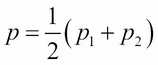We can now write the decision boundary as the following: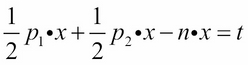Using the kernel trick, we can obtain the following decision boundary: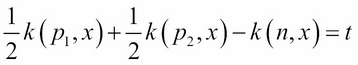With the kernel we defined earlier, we get the following: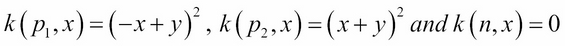We can now derive the decision boundary: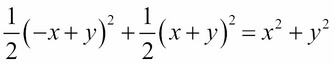This is simply a circle around the origin with a radius √t.

Using the kernel trick, on the other hand, each new instance is evaluated against each training example. In return for this more complex calculation we obtain a more flexible non-linear decision boundary.

A very interesting and important aspect is the interaction between features. One form of interaction is correlation. For example, words in a blog post, where we might perhaps expect there to be a positive correlation between the words winter and cold, and a negative correlation between winter and hot. What this means for your model depends on your task. If you are doing a sentiment analysis, you might want to consider reducing the weights of each word if they appear together since the addition of another correlated word would be expected to contribute marginally less weight to the overall result than if that word appeared by itself.

Also with regards to sentiment analysis, we often need to transform certain features to capture their meaning. For example, the phrase not happy contains a word that would, if we just used 1-grams, contribute to a positive sentiment score even though its sentiment is clearly negative. A solution (apart from using 2-grams, which may unnecessarily complicate the model) would be to recognize when these two words appear in a sequence and create a new feature, not_happy, with an associated sentiment score.

Selecting and optimizing features is time well spent. It can be a significant part of the design of learning systems. This iterative nature of design flips between two phases. Firstly, understanding the properties of the phenomena you are studying, and secondly, testing your ideas with experimentation. This experimentation gives us deeper insight into the phenomena, allowing us to optimize our features and gain deeper understanding, among other things, until we are satisfied about our model giving us an accurate reflection of reality.

### Unified modeling language

Machine learning systems can be complex. It is often difficult for a human brain to understand all the interactions of a complete system. We need some way to abstract the system into a set of discrete functional components. This enables us to visualize our system's structure and behavior with diagrams and plots.

UML is a formalism that allows us to visualize and communicate our design ideas in a precise way. We implement our systems in code, and the underlying principles are expressed in mathematics, but there is a third aspect, which is, in a sense, perpendicular to these, and that is a visual representation of our system. The process of drawing out your design helps conceptualize it from a different perspective. Perhaps we could consider trying to triangulate a solution.

Conceptual models are theoretical devices for describing elements of a problem. They can help us clarify assumptions, prove certain properties, and give us a fundamental understanding of the structures and interactions of systems.

UML arose out of the need to both simplify this complexity and allow our designs to be communicated clearly and unambiguously to team members, clients, and other stakeholders. A model is a simplified representation of a real system. Here, we use the word model in a more general sense, as compared to its more precise machine learning definition. UML can be used to model almost any system imaginable. The core idea is to strip away any irrelevant and potentially confusing elements with a clear representation of core attributes and functions.

#### Class diagrams

The class diagram models the static structure of a system. Classes represent abstract entities with common characteristics. They are useful because they express, and enforce, an object-oriented approach to our programming. We can see that by separating distinct objects in our code, we can work more clearly on each object as a self-contained unit. We can define it with a specific set of characteristics, and define how it relates to other objects. This enables complex programs to be broken down into separate functional components. It also allows us to subclass objects via inheritance. This is extremely useful and mirrors how we model the particularly hierarchical aspect of our world (that is, programmer is a subclass of human, and Python programmer is a subclass of programmer). Object programming can speed up the overall development time because it allows the reuse of components. There is a rich class library of developed components to draw upon. Also, the code produced tends to be easier to maintain because we can replace or change classes and are able to (usually) understand how this will affect the overall system.

In truth, object coding does tend to result in a larger code base, and this can mean that programs will be slower to run. In the end, it is not an "either, or" situation. For many simple tasks, you probably do not want to spend the time creating a class if you may never use it again. In general, if you find yourself typing the same bits of code, or creating the same type of data structures, it is probably a good idea to create a class. The big advantage of object programming is that we can encapsulate the data and the functions that operate on the data in one object. These software objects can correspond in quite a direct way with real world objects.

Designing object-oriented systems may take some time, initially. However, while establishing a workable class structure and class definitions, the coding tasks required to implement the class becomes clearer. Creating a class structure can be a very useful way to begin modeling a system. When we define a class, we are interested in a specific set of attributes, as a subset of all possible attributes or actual irrelevant attributes. It should be an accurate representation of a real system, and we need to make the judgment as to what is relevant and what is not. This is difficult because real world phenomena are complex, and the information we have about the system is always incomplete. We can only go by what we know, so our domain knowledge (the understanding of the system(s) we are trying to model), whether it be a software, natural, or human, is critically important.

#### Object diagrams

Object diagrams are a logical view of the system at runtime. They are a snapshot at a particular instant in time and can be understood as an instance of a class diagram. Many parameters and variables change value as the program is run, and the object diagram's function is to map these. This runtime binding is one of the key things object diagrams represent. By using links to tie objects together, we can model a particular runtime configuration. Links between objects correspond to associations between the objects class. So, the link is bound by the same constraints as the class that it enforces on its object.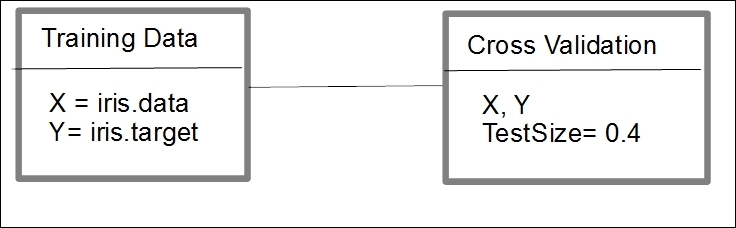The object diagram

Both, the class diagram and the object diagram, are made of the same basic elements. While the class diagram represents an abstract blueprint of the class. The object diagram represents the real state of an object at a particular point in time. A single-object diagram cannot represent every class instance, so when drawing these diagrams, we must confine ourselves to the important instances and instances that cover the basic functionality of the system. The object diagram should clarify the association between objects and indicate the values of important variables.

#### Activity diagrams

The purpose of an activity diagram is to model the system's work flow by chaining together separate actions that together represent a process. They are particularly good at modeling sets of coordinated tasks. Activity diagrams are one of the most used in the UML specification because they are intuitive to understand as their formats are based on traditional flow chart diagrams. The main components of an activity diagram are actions, edges (sometimes called paths) and decisions. Actions are represented by rounded rectangles, edges are represented by arrows, and decisions are represented by a diamond. Activity diagrams usually have a start node and an end node.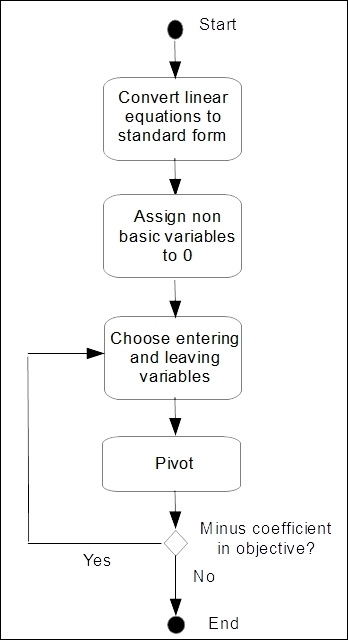A figure of an example activity diagram

#### State diagrams

State diagrams are used to model systems that change behavior depending on what state they are in. They are represented by states and transitions. States are represented by rounded rectangles and transitions by arrows. Each transition has a trigger, and this is written along the arrow.

Many state diagrams will include an initial pseudo state and a final state. Pseudo states are states that control the flow of traffic. Another example is the choice pseudo state. This indicates that a Boolean condition determines a transition.

A state transition system consists of four elements; they are as follows:

• S = {s1, s2, …}: A set of states

• A= {a1, a2, ...}: A set of actions

• E ={e1, e2, ...}: A set of events

• y: S(A U E)→2s: A state transition function

The first element, S, is the set of all possible states the world can be in. Actions are the things an agent can do to change the world. Events can happen in the world and are not under the control of an agent. The state transition function, y, takes two things as input: a state of the world and the union of actions and events. This gives us all the possible states as a result of applying a particular action or event.

Consider that we have a warehouse that stocks three items. We consider the warehouse only stocks, at most, one of each item. We can represent the possible states of the warehouse by the following matrix: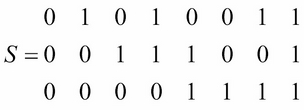This can define similar binary matrices for E, representing the event sold, and A, which is an action order.

In this simple example, our transition function is applied to an instance (s, which is a column in S), which is s' = s + a - e, where s' is the system's final state, s is its initial state, and a and e are an activity and an event respectively.

We can represent this with the following transition diagram: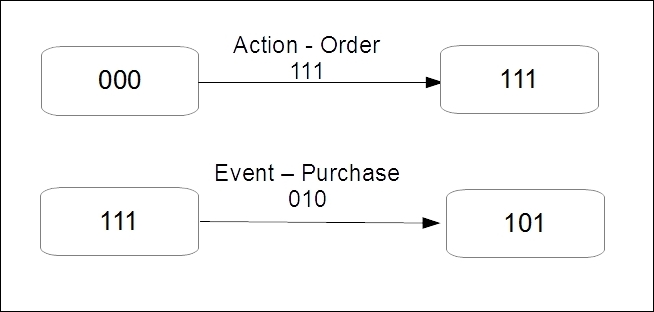The figure of a transition Diagram

## Summary

So far, we have introduced a broad cross-section of machine learning problems, techniques, and concepts. Hopefully by now, you have an idea of how to begin tackling a new and unique problem by breaking it up into its components. We have reviewed some of the essential mathematics and explored ways to visualize our designs. We can see that the same problem can have many different representations, and that each one may highlight different aspects. Before we can begin modeling, we need a well-defined objective, phrased as a specific, feasible, and meaningful question. We need to be clear how we can phrase the question in a way that a machine can understand.

The design process, although consisting of different and distinct activities, is not necessarily a linear process, but rather more of an iterative one. We cycle through each particular phase, proposing and testing ideas until we feel we can jump to the next phase. Sometimes we may jump back to a previous stage. We may sit at an equilibrium point, waiting for a particular event to occur; we may cycle through stages or go through several stages in parallel.

In the next chapter, we will begin our exploration of the practical tools that are available in the various Python libraries.

### About the Author

• ##### David Julian

David Julian is a freelance technology consultant and educator. He has worked as a consultant for government, private, and community organizations on a variety of projects, including using machine learning to detect insect outbreaks in controlled agricultural environments (Urban Ecological Systems Ltd., Bluesmart Farms), designing and implementing event management data systems (Sustainable Industry Expo, Lismore City Council), and designing multimedia interactive installations (Adelaide University). He has also written Designing Machine Learning Systems With Python for Packt Publishing and was a technical reviewer for Python Machine Learning and Hands-On Data Structures and Algorithms with Python - Second Edition, published by Packt.

Browse publications by this author

### Latest Reviews

(5 reviews total)